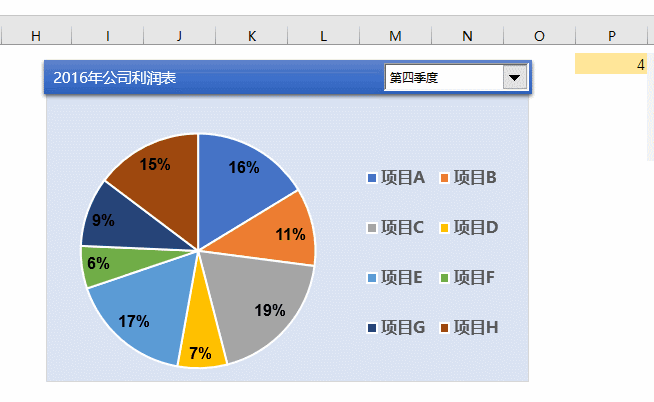# 动态数字货币交易策略回测平台-python1. 要访问binance API，只需从命令行pip install binance client。其他依赖项安装在其各自的导入行上注释。2. binance客户端请求API密钥时，这些密钥仅对于将交易订单实时发送到币安交易所是必需的，而对于读取数据则不是必需的。我保留了格式，以防这是任何读者所期望的用例。3. 在收集了每个代币的数据之后，我们需要让脚本停止运行60秒。这是为了防止binance API因为我们请求太多的数据而把我们踢出去。我目前没有遇到过这种问题，但如果遇到错误，这个时间可能需要增加。

4. 这里包括一个简单的移动平均函数，它展示了如何从价格数据中设计特性并将其添加到dataframe中的示例。这可以是您选择的自定义函数，也可以只是一个在网上找到的通用指示器的函数，如图所示。

5. 卷数据也可用，但在本例中未使用。如果您感兴趣，请将其添加到第32行。

6. csv文件的自定义命名可以在第72行的引号中编辑。

import numpy as np #pip install numpy

from tqdm import tqdm #pip install tqdm

from binance.client import Client #pip install python-binance

import pandas as pd #pip install pandas

from datetime import datetime

import random

SMA_LOW = 40

SMA_HIGH = 150

def compute_sma(data, window):

sma = data.rolling(window=window).mean()

return sma

#select cryptocurrencies you'd like to gather and time interval

START_TIME = '28 Mar, 2019'

END_TIME = '1 Jun, 2020'

api_key=''

api_secret=''

client = Client(api_key=api_key,api_secret=api_secret)

merge = False

for ratio in ratios:

print(f'Gathering data...')

data = client.get_historical_klines(symbol=f'USDT',interval=Client.KLINE_INTERVAL_1MINUTE,start_str=START_TIME,end_str=END_TIME)

temp_df = pd.DataFrame(data,columns=cols)

temp_df = temp_df[['time',f'-USD_close']]

if merge == False:

df = temp_df

else:

df = pd.merge(df,temp_df,how='inner',on='time')

merge = True

print('complete')

time.sleep(60) #sleep for a bit so the binance api doesn't kick you out for too many data asks

for col in df.columns:

if col != 'time':

df[col] = df[col].astype(np.float64)

for ratio in ratios:

df[f'_'] = compute_sma(df[f'-USD_close'], SMA_LOW)

df[f'_'] = compute_sma(df[f'-USD_close'], SMA_HIGH)

#clip NaNs

df = df[SMA_HIGH:]

df = df.reset_index(drop=True)

#convert binance timestamp to datetime

for i in tqdm(range(len(df))):

df['time'][i] = datetime.fromtimestamp(int(df['time'][i]/1000))

df.to_csv('12-coins-Mar18_Jun20')Memory

class Memory:

def __init__(self):

self.clear()

def clear(self):

self.actions = []

self.actions.append(new_action)

Env.step()方法

DAYS_PER_EPISODE = 1

MINUTES_PER_EPISODE = 1440*DAYS_PER_EPISODE

NUM_EPISODES = 100

TRADING_FEE_MULTIPLIER = .99925 #this is the trading fee on binance VIP level 0 if using BNB to pay fees

class Env:

def __init__(self, ratios, df):

self.ratios = ratios

self.main_df = df

self.reset()

def reset(self):

self.balances = {'USD':1.0}

for ratio in self.ratios:

self.balances[ratio] = .

self.iloc = random.randint(,len(self.main_df)-MINUTES_PER_EPISODE-1)

self.episode_df = self.main_df[self.iloc:self.iloc+MINUTES_PER_EPISODE+2]

self.money_in = 'USD'

self.start_time = self.episode_df['time'].iloc[]

self.end_time = self.episode_df['time'].iloc[-1]

def step(self):

self.iloc+=1

#-------IMPLEMENT STRATEGY HERE--------

if self.money_in == 'USD':

for ratio in self.ratios:

#if low sma crosses above high sma

if self.episode_df[f'_'][self.iloc] > self.episode_df[f'_'][self.iloc] and self.episode_df[f'_'][self.iloc-1] > self.episode_df[f'_'][self.iloc-1]:

self.balances['USD'] = .

break

if self.money_in != 'USD': #can't sell if money_in usd

if self.episode_df[f'_'][self.iloc]

#if high sma crosses below low sma

#sell money_in/USD

self.balances[self.money_in] = .

self.sell_price = self.episode_df[f'-USD_close'][self.iloc]

self.money_in = 'USD'

#-------IMPLEMENT STRATEGY HERE--------

#-------CALCULATE PERFORMANCE METRICS HERE-------

#Running net worth

self.net_worth = self.balances['USD']

for ratio in self.ratios:

self.net_worth += self.balances[ratio]*self.episode_df[f'-USD_close'][self.iloc]

#Net_worth had you owned all ratios over episode_df --> 'average_market_change'

self.average_start_price =

self.average_end_price =

for ratio in self.ratios:

self.average_start_price += self.episode_df[f'-USD_close'].iloc[]

self.average_end_price += self.episode_df[f'-USD_close'].iloc[-1]

self.average_start_price /= len(ratios)

self.average_end_price /= len(ratios)

self.average_market_change = self.average_start_price / self.average_end_price

#-------CALCULATE PERFORMANCE METRICS HERE-------

return self.net_worth, self.average_market_change, self.start_time, self.end_time

env = Env(ratios, df)

memory = Memory()

net_worth_collect = []

average_market_change_collect = []

for i_episode in range(NUM_EPISODES):

for i in range(len(env.episode_df)-1):

net_worth, average_market_change, start_time, end_time = env.step()

net_worth_collect.append(net_worth)

average_market_change_collect.append(average_market_change)

#log after each episode

print(f'episode: ')

print(memory.actions)

print('\n')

print(f'interval: - ')

print(f'net worth after day(s): ')

print(f'average market change: ')

print('\n')

memory.clear()

env.reset()

#log overall

print(f'net worth average after backtest episodes: ')

#Yes, average of the average market changes

print(f'average, average market change over episodes: ')

episode: 96

interval: 2019-04-29 12:25:00 - 2019-04-30 12:26:00

net worth after 1 day(s): 1.0221672847272814

average market change: 0.988959335567251

episode: 99

interval: 2019-07-28 16:39:00 - 2019-07-29 16:40:00

net worth after 1 day(s): 0.9218801235080804

average market change: 0.9984598063740531

net worth average after 100 backtest episodes: 0.9656146271760888

average, average market change over 100 episodes: 1.0021440510159623

python日志记录包在这里对于运行许多不同策略并登录到单独文件以比较结果的人可能有用。

-------------------------------------

-------------------------------------